Learn all Concepts of Polynomials Class 9 (with VIDEOS). Check - Polynomials Class 9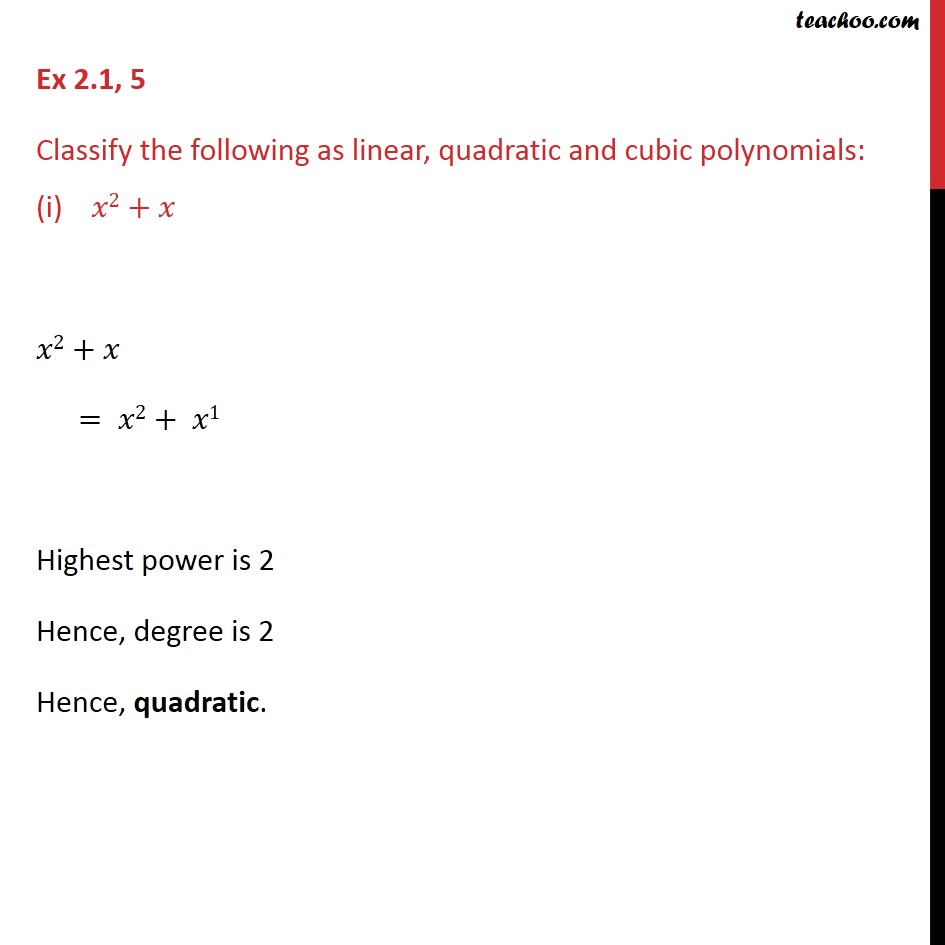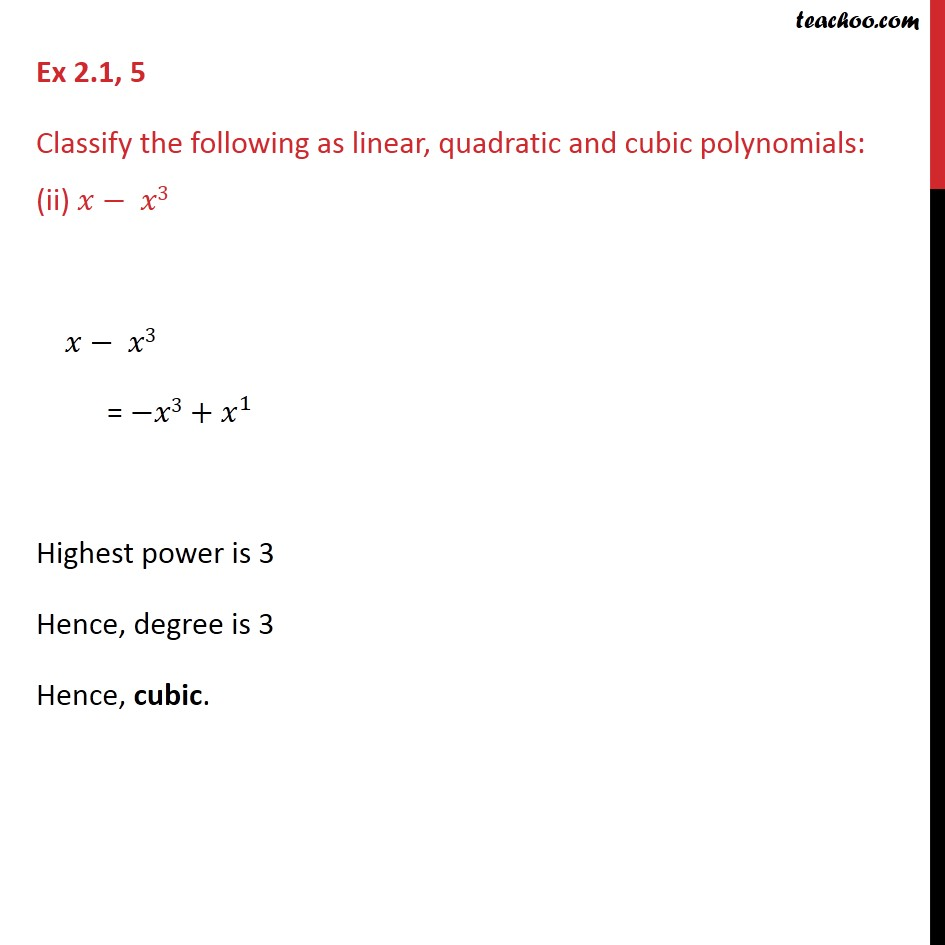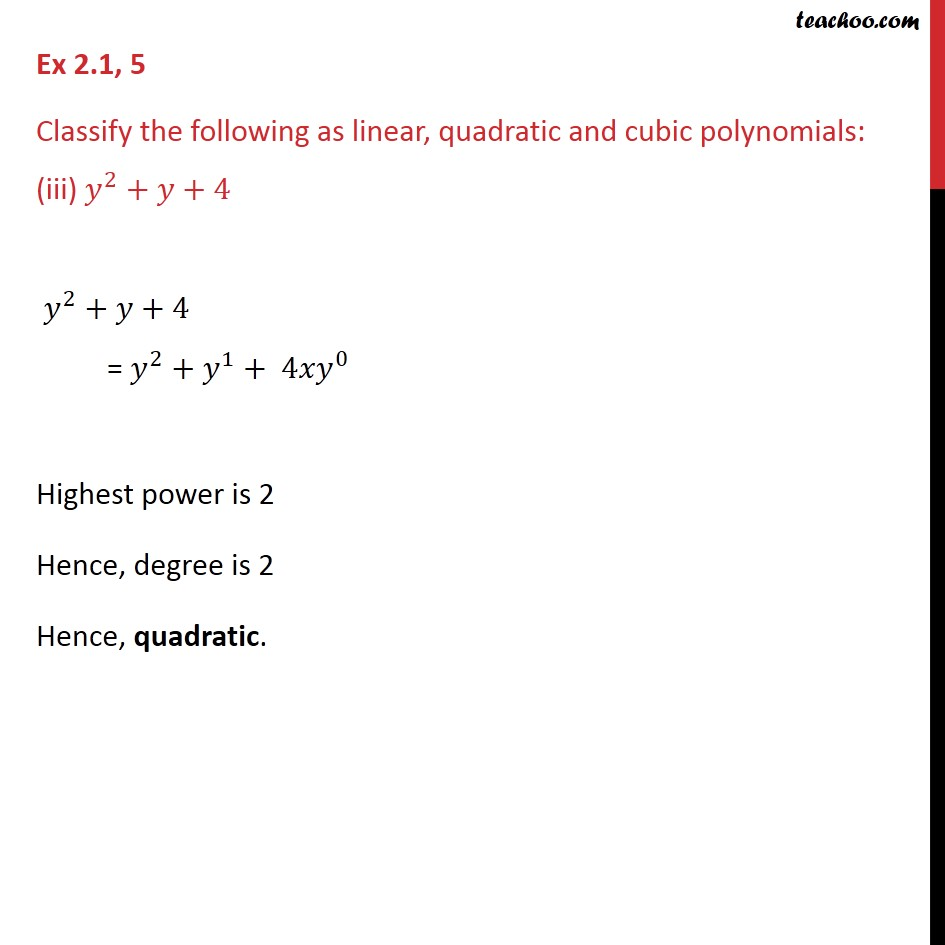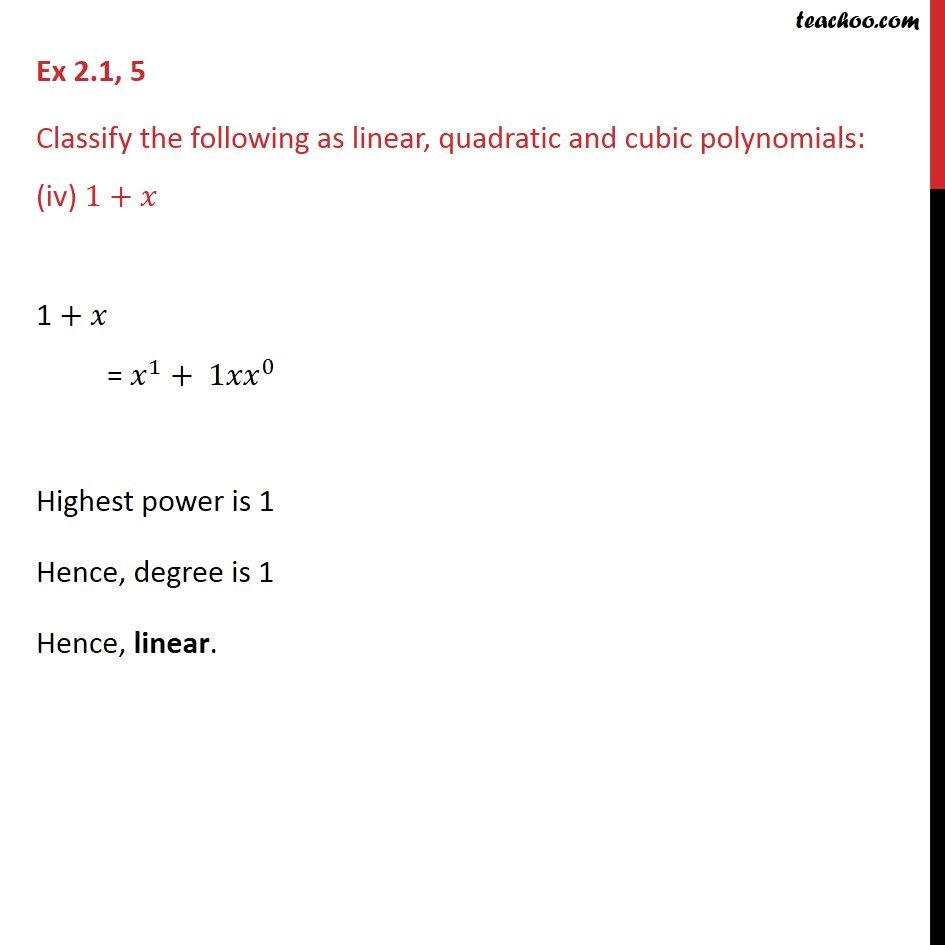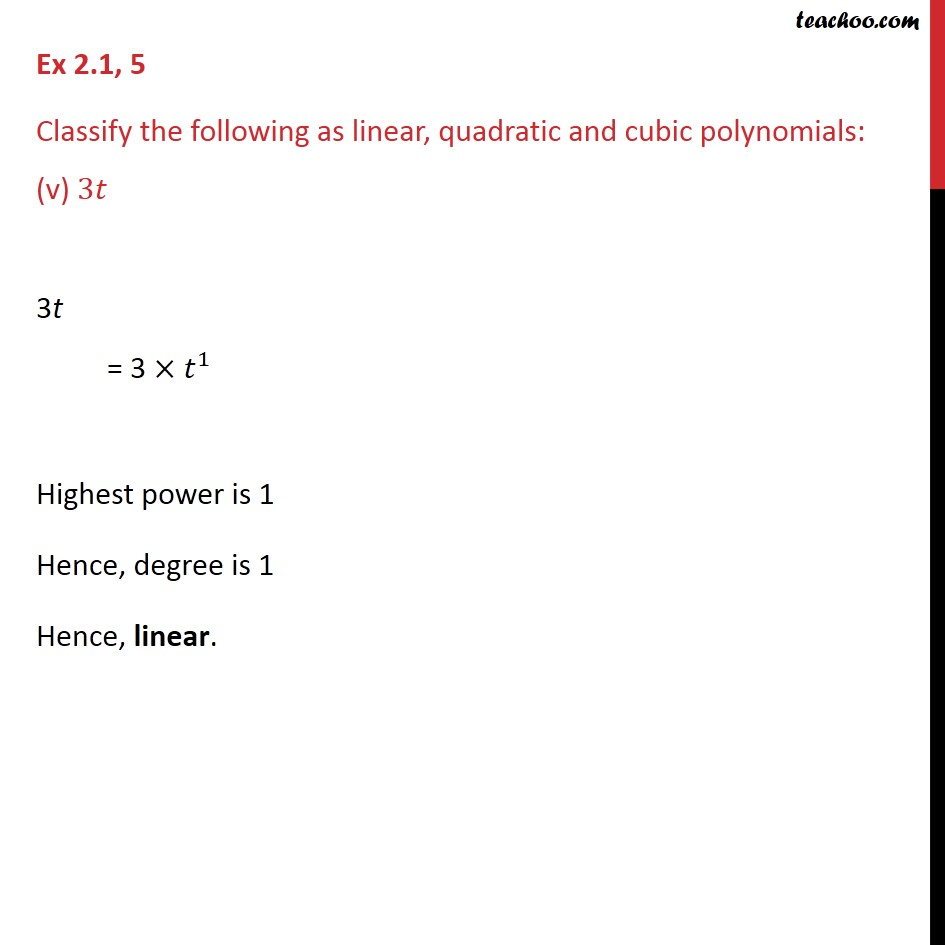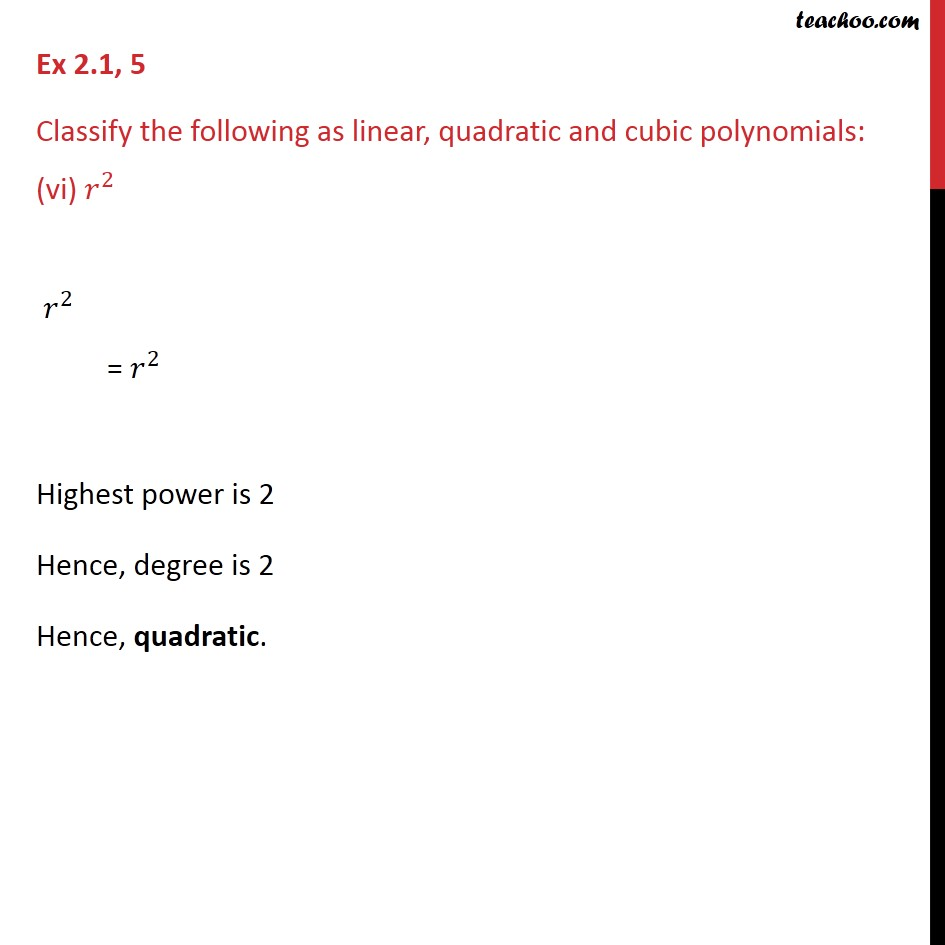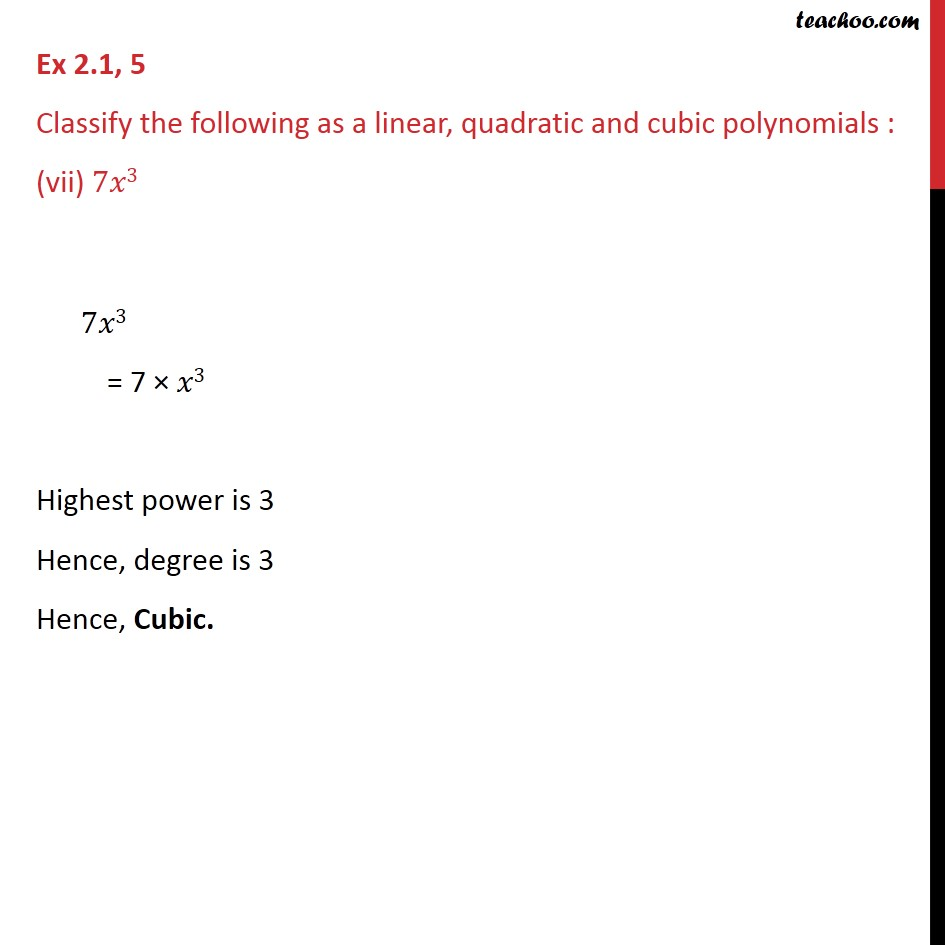1. Chapter 2 Class 9 Polynomials
2. Serial order wise
3. Ex 2.1

Transcript

Ex 2.1, 5 Classify the following as linear, quadratic and cubic polynomials: (i) 2+ 2+ = 2+ 1 Highest power is 2 Hence, degree is 2 Hence, quadratic. Ex 2.1, 5 Classify the following as linear, quadratic and cubic polynomials: (ii) 3 3 = 3 + 1 Highest power is 3 Hence, degree is 3 Hence, cubic. Ex 2.1, 5 Classify the following as linear, quadratic and cubic polynomials: (iii) 2 + +4 2 + +4 = 2 + 1 + 4 0 Highest power is 2 Hence, degree is 2 Hence, quadratic. Ex 2.1, 5 Classify the following as linear, quadratic and cubic polynomials: (iv) 1+ 1 + = 1 + 1 0 Highest power is 1 Hence, degree is 1 Hence, linear. Ex 2.1, 5 Classify the following as linear, quadratic and cubic polynomials: (v) 3 3t = 3 1 Highest power is 1 Hence, degree is 1 Hence, linear. Ex 2.1, 5 Classify the following as linear, quadratic and cubic polynomials: (vi) 2 2 = 2 Highest power is 2 Hence, degree is 2 Hence, quadratic. Ex 2.1, 5 Classify the following as linear, quadratic and cubic polynomials: (vii) 2 7 2 = 7 3 Highest power is 3 Hence, degree is 3 Hence, cubic.

Ex 2.1

Chapter 2 Class 9 Polynomials
Serial order wise